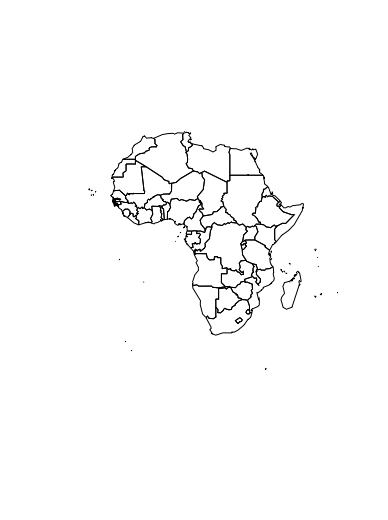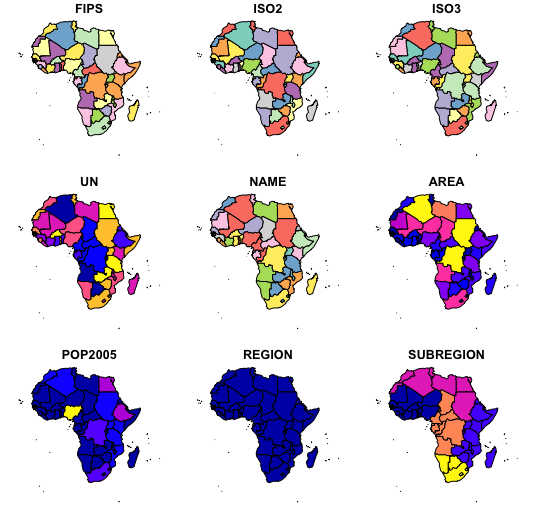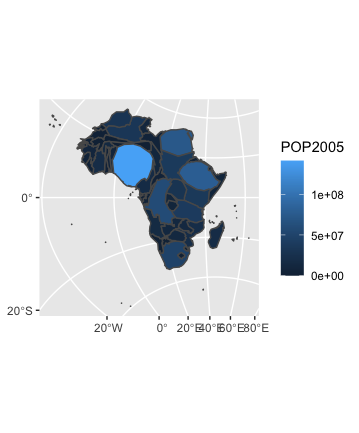# Basic Cartogram with R

A cartogram is a map in which the geometry of regions is distorted in order to convey the information of an alternate variable. In this post, we are going to draw a map of Africa where the size of each country is distorted proportionally to its population. A cartogram is frequently a choropleth map in which regions are colored according to a numeric value (not necessarily the one used to build the cartogram). To understand the cartogram more easily, one can refer to what a geospatial object is.

## Preparing the Map data and creating Basic Cartogram

To perform a cartogram map we will create map data using wrld_simpl.

## R

 `# library for the map data` `library``(maptools) ` `data``(wrld_simpl)`   `# extracting the African map data ` `# this data contains the latitude and longitude of ` `# region boundaries and regions` `afr = wrld_simpl[wrld_simpl\$REGION==2,]`   `# plotting outline map` `plot``(afr)`

Output:## Spatial Polygon Features in Cartogram

We have a spatial object and a numeric value associated with each region, it is possible to color each region according to its value.

## R

 `library``(sf) ``# library to get the SpatialPolygon` `afr_sf = ``st_as_sf``(afr)` `plot``(afr_sf)`

Output:### Cartogram and its customization

Here we will create cartogram and then we will customize for this we will use wrld_simpl and then extract the information like shape, coordinates and then transform geometry polygon objects to new geospatial objects.

## R

 `library``(tidyverse)`   `# Get the shape file of Africa` `library``(maptools)`   `# retrieve the data for the countries` `data``(wrld_simpl)`   `# extract the data for Africa` `# afr_data holds the data about the African polygon shape` `# and information like coordinates, latitude and longitude` `afr_data = wrld_simpl[wrld_simpl\$REGION == 2,]` `cat ``(``"Type of afr_data: "``, ``typeof``(afr_data), ``"\n\n"``)`   `# We work with the cartogram library ` `library``(cartogram)`   `# s4 is an system for object oriented programming` `# convert this foreign object to sf object` `sfno = ``st_as_sf``(afr)` `# transform geometry polygon objects to a new coordinate reference system ` `sfnoproj = ``st_transform``(sfno, crs = 23038)`   `# construct a cartogram using the population in 2005` `afr_cartogram <- ``cartogram_cont``(sfnoproj, ``"POP2005"``, itermax = 7)`   `# This is a new geospatial object, we can visualise it!` `ggplot``() +` `  ``geom_sf``(data = afr_cartogram, ``aes``(fill = POP2005))`

Output:

```Type of afr_data:  S4
Mean size error for iteration 1: 6.65362274402649
Mean size error for iteration 2: 5.77932593731838
Mean size error for iteration 3: 5.11349284302375
Mean size error for iteration 4: 4.56333360251358
Mean size error for iteration 5: 4.09786807153034
Mean size error for iteration 6: 3.69769020137334
Mean size error for iteration 7: 3.3519304681889```Whether you're preparing for your first job interview or aiming to upskill in this ever-evolving tech landscape, GeeksforGeeks Courses are your key to success. We provide top-quality content at affordable prices, all geared towards accelerating your growth in a time-bound manner. Join the millions we've already empowered, and we're here to do the same for you. Don't miss out - check it out now!

Previous
Next